# The Quadratic formula

##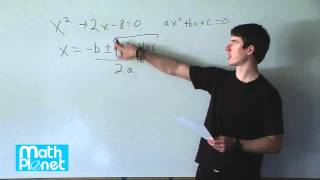By MathPlanetVideos

Solve the given equation using the quadratic formula x2+2x−8=0# Using the quadratic formula

##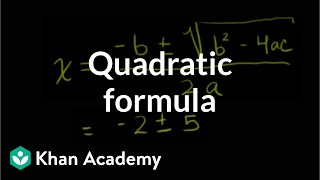By Khan Academy

Sal introduces the quadratic formula and how it should be used in order to solve quadratic equations.# Using the quadratic formula

##By Khan Academy

Sal introduces the quadratic formula and how it should be used in order to solve quadratic equations.# Using the quadratic formula

##By Khan Academy

Sal introduces the quadratic formula and how it should be used in order to solve quadratic equations.# Example 2: Using the quadratic formula | Quadratic equations | Algebra I | Khan Academy

##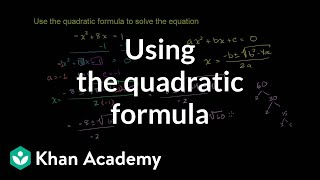By Khan Academy

U10_L1_T3_we2 Quadratic Formula 2# Solving Quadratic Equations using the Quadratic Formula - Example 2, Complex Solutions

##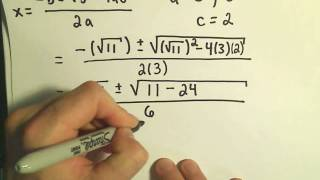By PatrickJMT

YouTube presents Solving Quadratic Equations using the Quadratic Formula - Example 2, Complex Solutions an educational video resources on math.# Solving Quadratic Equations using the Quadratic Formula - Example 2, Complex Solutions

##By PatrickJMT

YouTube presents Solving Quadratic Equations using the Quadratic Formula - Example 2, Complex Solutions an educational video resources on math.# Using the quadratic formula

##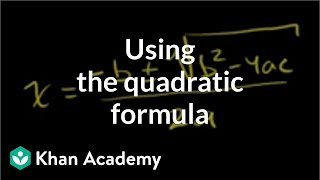By Khan Academy

Sal solves the equation -7q^2+2q+9=0 by using the quadratic formula.# Using the quadratic formula

##By Khan Academy

Sal solves the equation -x^2+8x=1 by first bringing it to standard form and the using the quadratic formula.# Using the quadratic formula

##By Khan Academy

Sal solves the equation -x^2+8x=1 by first bringing it to standard form and the using the quadratic formula.# Using the quadratic formula

##By Khan Academy

Sal solves the equation -x^2+8x=1 by first bringing it to standard form and the using the quadratic formula.# Using the quadratic formula

##By Khan Academy

Sal solves the equation -7q^2+2q+9=0 by using the quadratic formula.# Using the quadratic formula

##By Khan Academy

Sal solves the equation -7q^2+2q+9=0 by using the quadratic formula.# Quadratic formula (proof) | Quadratic equations | Algebra I | Khan Academy

##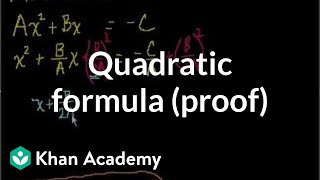By Khan Academy

Watch this video to learn how the quadratic formula is proven from completing the square for the general quadratic equation Ax2 + Bx + C = 0.# Using the quadratic formula

##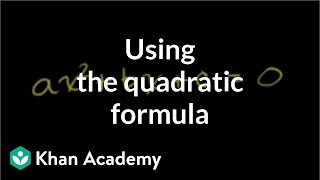By Khan Academy

Sal rewrites the equation 6x^2+3=2x-6 in standard form and identifies a, b, and c, that can later be used within the quadratic formula.# Using the quadratic formula

##By Khan Academy

Sal rewrites the equation 6x^2+3=2x-6 in standard form and identifies a, b, and c, that can later be used within the quadratic formula.# Using the quadratic formula

##By Khan Academy

Sal rewrites the equation 6x^2+3=2x-6 in standard form and identifies a, b, and c, that can later be used within the quadratic formula.# Example 5: Using the quadratic formula | Quadratic equations | Algebra I | Khan Academy

##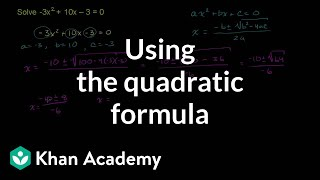By Khan Academy

Applying the Quadratic Formula-From Khan Academy# Example 1: Using the quadratic formula | Quadratic equations | Algebra I | Khan Academy

##By Khan Academy

This video from Khan Academy introduces the quadratic formula, and includes illustrative examples of its application.# Proof of quadratic formula | Polynomial and rational functions | Algebra II | Khan Academy

##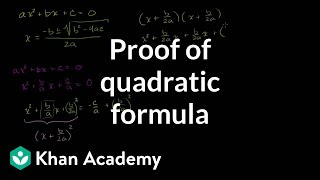By Khan Academy

This instructor in this video, Sal Khan, in an understandable manner, discusses how to prove a quadratic formula. Mr. Khan uses computer software (with different colors) to illustrate his points. Sal Khan is the recipient of the 2009 Microsoft Tech Award in Education. The viewer may want to open the video to 'full screen' as the instructor fits a lot of information on a dark screen.